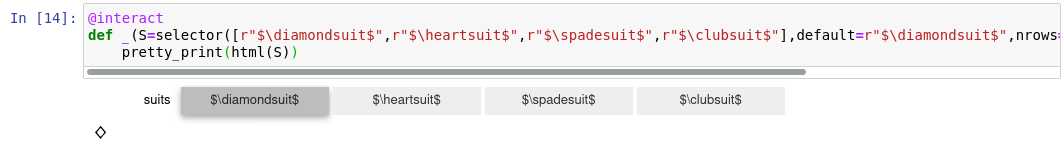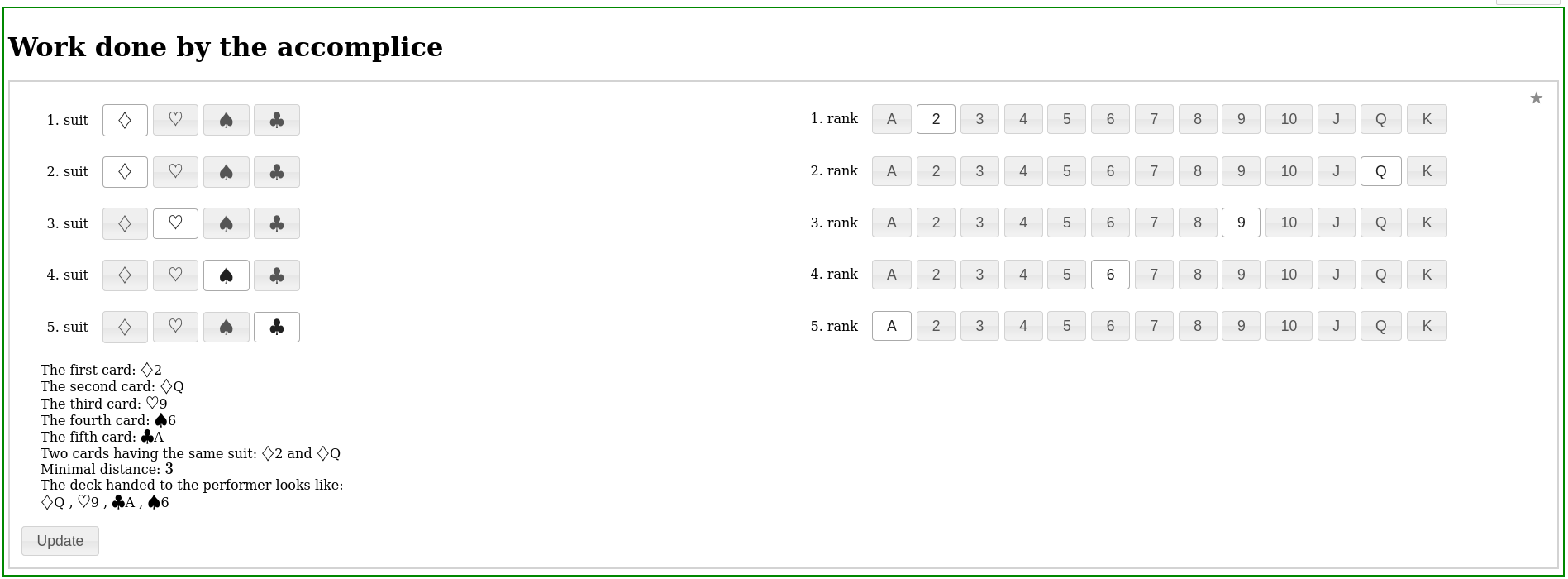# Revision history [back]

### Interact selector containing card suits

I have the following code that used to work in the Python 2 era:

@interact
def _(S=selector([r"$\diamondsuit$",r"$\heartsuit$",r"$\spadesuit$",r"$\clubsuit$"],default=r"$\diamondsuit$",nrows=1,buttons=True,label="suits")):
pretty_print(html(S))


Now it provides. Is there a way to have the expected symbols in the selector? Thank you for your suggestions in advance.

### Interact selector containing card suits

I have the following code that used to work in the Python 2 era:

@interact
def _(S=selector([r"$\diamondsuit$",r"$\heartsuit$",r"$\spadesuit$",r"$\clubsuit$"],default=r"$\diamondsuit$",nrows=1,buttons=True,label="suits")):
pretty_print(html(S))


Now it provides. Is there a way to have the expected symbols in the selector? Thank you for your suggestions in advance.

OK, it seems to work online using SageMath Cell, in case of Jupyter Notebook I still do not know an answer.